# SSC Quantitative Aptitude Questions (Day-76)

Dear Aspirants, you can find the Quantitative Aptitude questions with detailed explanations for the SSC exams. Nowadays the competitive level of the exam has been increasing consistently. Due to the great demand for the government job, the level of the toughness reached greater. Candidates have to enhance the preparation process in order to drive in the right path. It doesn’t need to clear the prescribed cutoff. You must have to score good marks more than the cut off marks to get into the final provisional list. Here we have updating the Quantitative Aptitude questions with detailed explanations on a daily basis. You can practice with us and measure your level of preparation. According to that you can sculpt yourself in a proper way. SSC aspirants kindly make use of it and grab your success in your career.

Start Quiz

1) If interest being compound annually then what sum will amount to Rs 2508.8 in 2 years at the rate of 12% per annum at compound interest?

(a) 2000

(b) 2200

(c) 1800

(d) 2500

Direction (2-5): The line chart given below shows the revenue of a company (in lac rupees) from year 2009 to 2016.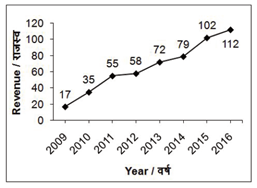2) The revenue in year 2013 is what percent of the revenue in year 2011?

(a) 30.9

(b) 137.2

(c) 130.9

(d) 45.9

3) In how many years from 2010 to 2016 the increase in the revenue is more than 20% of the previous year value?

(a) 3

(b) 4

(c) 5

(d) 6

4) The average revenue of the company from 2013 to 2016 is how much percent more than the average revenue of the company from 2009 to 2012?

(a) 181.2

(b) 21.2

(c) 121.2

(d) 221.2

5) If from year 2007 to year 2009 the revenue has decreased by 20% each year, then what was the revenue (in lac Rs) of the company in year 2007?

(a) 26.56

(b) 21.56

(c) 29.52

(d) 31.56

6) The area of a rectangle is equal to the area of a square. If the area of the rectangle is 196 cm2, then what is the perimeter (in cm) of the square?

(a) 116

(b) 56

(c) 112

(d) 42

7) A cylinder having base radius equal to its height, right circular cone whose radius is equal to its height and a hemisphere are given. All of them have the same volumes. What will be the ratio of their respective radius?

(a) ∛3:1 : ∛2

(b) ∛2  : ∛2

(c) ∛2 : ∛6: ∛3

(d) ∛4 : ∛6 : √(2&3)

8) The perimeter of base of cylinder is 20π cm. If the height of cylinder is 12 cm, then what will be its curved surface area (in cm2)?

(a) 220π

(b) 180π

(c) 250π

(d) 240π

9) Side of the square base of a pyramid is 8 cm. The pyramid is cut in two parts by a plane parallel to its base such that heights of upper and lower parts are in ratio 1 : 3 respectively. What is the ratio of volume of lower and upper parts respectively?

(a) 27 : 1

(b) 63 : 1

(c) 64 : 1

(d) 26 : 1

10) There is a rectangular garden of 480 metre × 160 metre. A path of width 3 metre is build outside the garden. What is the area (in metre2) of the path?

(a) 3984

(b) 1892

(c) 2796

(d) 3876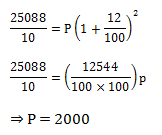72/55 × 100 = 130.9%

2010 → 17 + 3.4 = 20.4

2011 → 35 + 7 = 42

2012 → 55+ 11 = 66

2013 → 58 + 11.6 = 69.6

2014 → 72 + 14.4 = 86.4

2015 → 79 + 15.8 = 94.8

2016 → 102 + 20.4 = 122.4

So, (4)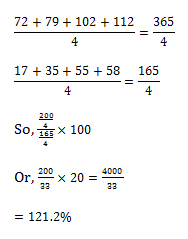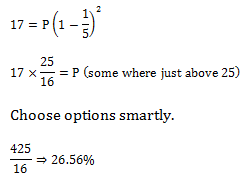196 = a2 ⇒ a = 14 cm

So, 4a = 56 cm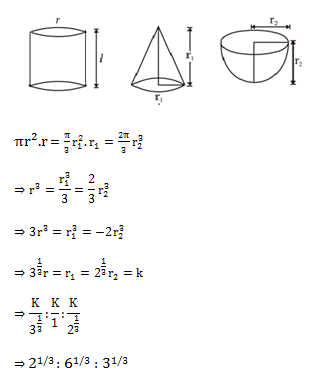20 π = 2πr

Or, r = 10 cm & h = 12 cm

∴ CSA = 20π × 12 = 240 π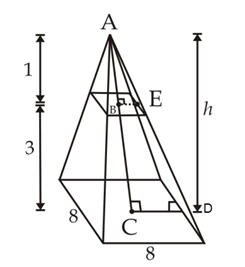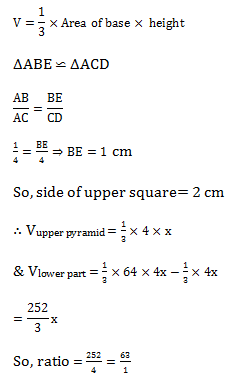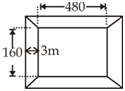3× (160 + 6) × 2 + 3 × (480 + 6) × 2 -9 × 4

= 6(166 + 486) -36

= 6(652) – 36

= 3912 – 36

= 3876 cm²

 Check Here to View SSC CGL / CHSL 2021 Quantitative Aptitude Questions Day – 75 Day – 74 Day – 73